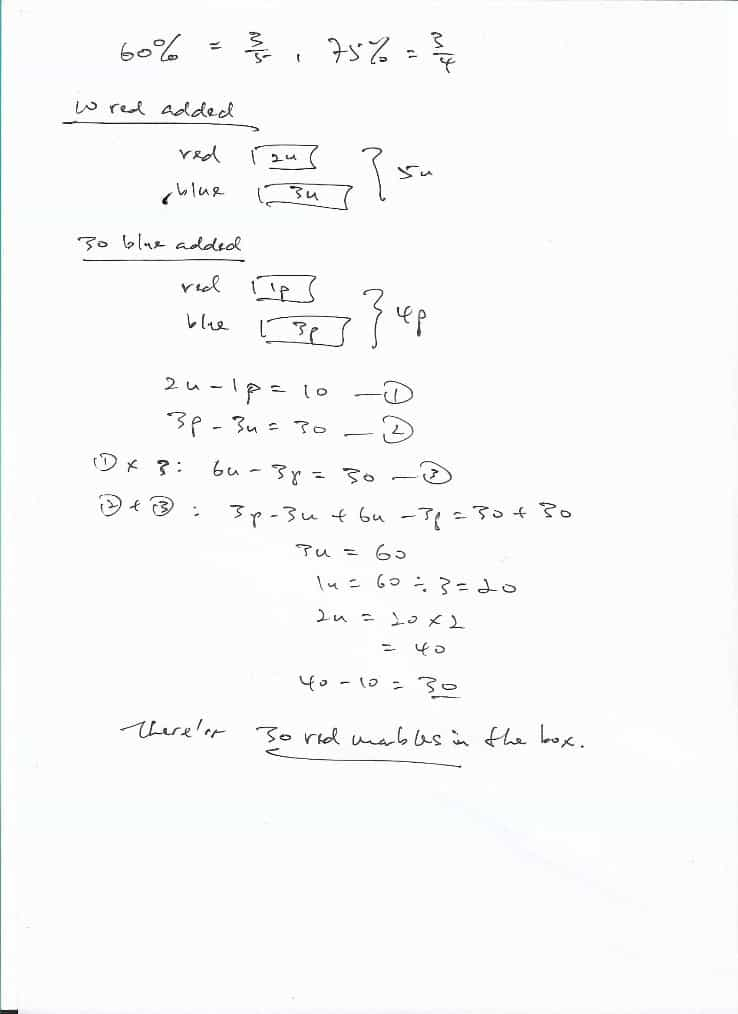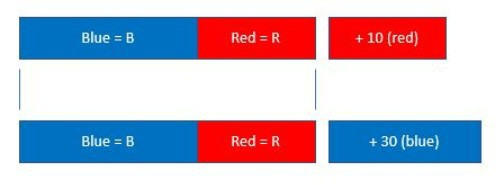# Question

A box contains some red and blue marbles. If Angeline adds in 10 red marbles, 60% of the marbles are blue. If she adds in 30 blue marbles, 75% of the marbles are blue. How many red marbles are there in the box?0 Replies 0 LikesLet number of blue & red marbles be B & R respectively.

First scenario:

Total number of Blue = B

Total number of Red = R + 10

Total number of marbles = B + R + 10

B / (B + R + 10) = 6/ 10 = 0.6

B = 0.6 (B + R + 10)

B = 0.6B + 0.6R + 6

0.4 B = 0.6R + 6

2B = 3R + 30 (both sides of equation x 5)

Second scenario:

Total number of Blue = B + 30

Total number of Red = R

Total number of marbles = B + R + 30

(B + 30) / (B + R + 30) = 75/100 = 0.75

B + 30 = 0.75 (B + R + 30)

B + 30 = 0.75B + 0.75R + 22.5

0.25B = 0.75R – 7.5

2B = 6R – 60 (both sides of equation x 8)

Therefore:

2B = 3R + 30 = 6R -60

3R = 90

R = 30

0 Replies 0 Likes